•••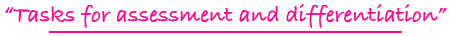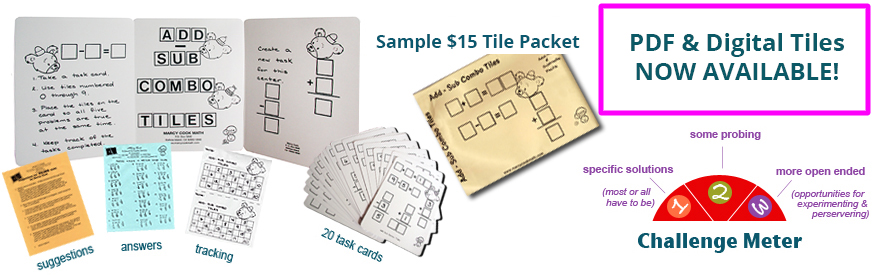Tiling Task Card Packets include 20 unique task cards on card stock,  3 backdrop cards , tracking sheets, suggestions and answer sheets.  These are contained in a printed manila envelope for convenient storing.  A set of number tiles 0 - 9 is  needed for each task card

NEW
4 - 6

### MULTIPLY TO A PRODUCT TILES

forming basic multiplication facts with 2 or 3 factors to a given product

Challenge Level: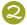AVAILABLE IN PDF FORMAT ONLY
(You will receive both color and black and white files for printing.)\$10.00

5 - 8

### MULTIPLY: THINK & TILE

forming a multiplication problem with a 4-digit number as a factor and a single-digit number as a factor by utilizing hints dealing with math concepts and vocabulary

Challenge Level: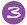\$15.00

5 - 8

### MULTIPLY: TRIAL & TILE

forming a multiplication problem where a 3-digit number is multiplied by a 2-digit number

Challenge Level:\$15.00

4 - 8

### NEGATIVE/POSITIVE INTEGER TILES

adding and subtracting of positive and negative whole numbers

Challenge Level:\$15.00

2 - 5

### NUMBER SENSE TILES

forming specified numbers using math vocabulary and concepts

Challenge Level:\$15.00

3 - 6

### NUMBER SEQUENCE TILES

sequencing numbers with patterns of plus, minus, or multiples in ascending and descending order

Challenge Level:\$15.00

4 - 8

### NUMBER SQUARED TILES

squaring the first ten counting numbers; pictorial and abstract

Challenge Level:\$15.00

2 - 4

### ODD & EVEN PICTURED TILES

recognizing even and odd 2-digit numbers from grid pictures and forming 2-digit even/odd numbers

Challenge Level: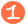\$15.00

4 - 8

### OPERATION TILES

forming equations using the 4 basic operations: addition, subtraction, multiplication, division

Challenge Level:\$15.00

5 - 8

### ORDER OF OPERATION TILES

utilizing the order of operations to form an equation

Challenge Level:\$15.00

1 - 3

### ORDER TILES

dealing with after, before, less than, more than, between, and numbers on a number line

Challenge Level:\$15.00

5 - 8

### ORDERED PAIR GRAPH TILES

reading x and y axis plotted points and figuring other ordered pairs which would fit the same linear equation

Challenge Level:\$15.00

1 - 3

### PARTLY PICTURED EQUATION TILES

forming balanced addition equations with the aid of pictures for the placement of some of the digits

Challenge Level:\$15.00

4 - 8

### PATTERN BLOCK RELATIONSHIP TILES

discovering the starting value of 1 of the pattern block shapes and completing relationship statements for the triangle,  blue rhombus, trapezoid, and hexagon

Challenge Level:\$15.00

2 - 5

### PATTERN TILES

forming addition problems with 3-digit addends and in column addition format where numerous solutions are possible; ideal for pursuit problem for the entire class

Challenge Level:\$15.00

5 - 8

### PERCENTAGE TILES I

changing common fractions to decimals and decimals to percents and vice versa

Challenge Level:\$15.00

5 - 8

### PERCENTAGE TILES II

computing the three basic types of percent problems

Challenge Level:\$15.00

3 - 6

### PICTURE FRACTION TILES

forming common fractions from pictures, including equivalent fractions and geometry vocabulary for part of a total group

Challenge Level:\$15.00

2 - 4

### PICTURE LOGIC TILES

observing and analyzing a given if...then...statement and applying that knowledge to the other pictured examples

Challenge Level:\$15.00

1 - 2

### PICTURE PLUS TILES

forming basic addition fact problems/solutions from pictured information; representational level activities

Challenge Level:\$15.00